# Analysis: Sequential-averaging components of the emissions boost factor

The temperature-variation emissions boost factor,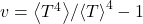, is a quantity that emerges as a result of averaging.

In practice, averaging is likely to be done in a variety of ways:

• over various time windows, such as day, month, season, or year
• over longitude (a “zonal” average), or globally (over both longitude and latitude)

Various types of averaging may be done in sequence.

So, it will potentially be useful to be able to talk about the contributions to the emissions boost factor that are associated with each averaging operation.

## Setup

Suppose quantities of interest are subject to a sequence of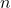averaging processes. Denote averaging process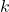of quantity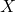by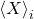. Also, denote the composite process of averagesthrough:

(1)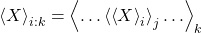It will be convenient to us use the notation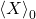to refer to the unaveraged value of.

## Decomposition A

Define:

(2)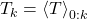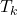is the temperature as averaged by the firstaveraging processes. Also, define:

(3)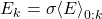where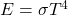and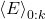is the value of this as averaged by the firstaveraging processes. Then, define:

(4)and take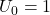. Basically,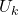isas calculated after the firstaveraging operations, and then with the value of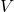averaged. Note that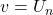.

We can then define:

(5)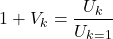It follows that:

(6)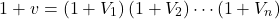so we can interpret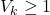as the contribution of averaging numberto the total value of.

## Decomposition B

Define:

(7)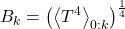If we take emissivityto be 1, or assume that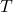is actually a surface “effective temperature” (i.e., “effective radiative emission temperature”) then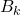can be interpreted as the “effective temperature” deduced from averaging the emitted radiative flux through the firstaveraging processes. Note that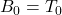and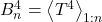.

Define:

(8)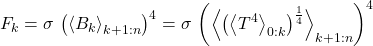Note thatand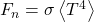.

We can expressin terms ofas follows:

(9)This can be written as:

(10)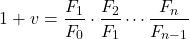(11)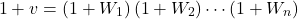where

(12)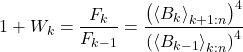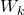is always greater than or equal to 0.

## Decomposition C

Define:

(13)is the temperature as averaged by the firstaveraging processes. Also, define:

(14)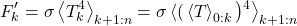Note that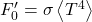and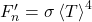.

The temperature-variation emissions boost factor,, is given by:

(15)This can be written as:

(16)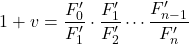(17)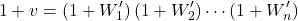where

(18)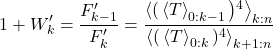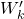is always greater than or equal to 1.

## Discussion

We’ve developed three distinct ways of decomposing a series of successive averages.

Decomposition A assumes we have data for bothand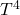which is well-resolved both geographically and temporally. Given that well-resolved information, it offers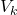as the contribution associated with that level of temperature non-uniformity.

Decompositions B and C assume that up until some level of aggregation, we only have accurate information about either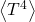(in the case of decomposition B) or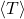(in the case of decomposition C), but not both. It’s assumed that at that point one tries to infer the value of the unknown quantity from the known quantity. The decompositions into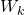or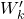indicate the loss in accurate estimation ofthat occurs if one aggregates through that level before beginning to work with separate averaging ofand.

These generic decomposition results can be applied to averaging over time and location, or over different time windows, or over different granularities of geographic location (e.g., regional, zonal, or global).